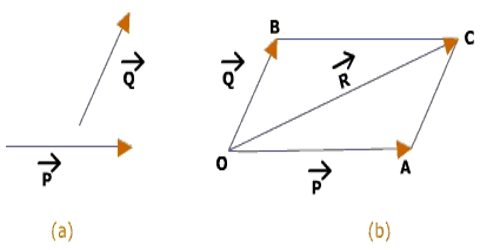# Parallelogram Law in Geometrical Addition of Vector Quantities

The Parallelogram Law

If two similar vectors acting simultaneously at a point can be represented both in magnitude and direction by two adjacent sides of a parallelogram, then the diagonal from the point of intersection of these sides gives the resultant vector both in magnitude and direction.Explanation: Let two vectors P and Q act simultaneously on a particle O at an angle α. Suppose, two vectors OA = P and OC = Q are acting at the same time at point O of a particle at an angle α (Fig.). Taking OA and OC as adjacent sides let us draw a parallelogram OABC and connect OB.

Now, according to the parallelogram law, the diagonal OB drawn from the tail-points of P and Q represents the resultant vector R;

OA + OC = OB

or, P + Q = R